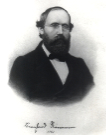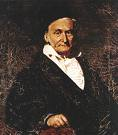### Contravariance and Covariance - Part 4Bernhard RiemannCarl Friedrich Gauss

Vector Concept 4 -- Vectors on Manifolds
Previously, our space was Rn ,which has a globaly defined rectangular coordinate system. We expand the spaces under consideration to include spaces more general than Euclidean space --manifolds. The discussion becomes somewhat more technical, and as a minimum, basic familiarity with point set topology is assumed.
Our underlying goal is apparently a rather modest one-- to generalize Rn and allow calculus to be done on this generalized space.
In some sense, mathematicians were already doing calculus on non-Euclidean spaces prior to the formal definition of a manifold, since surfaces such as S2, the sphere, are non-Euclidean. However, S2 is a subset of R3, but one would like to study surfaces (and in general, manifolds) intrinsically and not as subspaces of Rn. Gauss in fact initialized this method of investigation when he discovered the geometry of S2  could be ascertained from a study of its tangent vectors and their inner product
The modern notion of a manifold, which started with the researches of Gauss and Riemann, took many years to be clarified. To understand that the modern definition of a manifold is, in some sense, the correct generalization of Euclidean space, requires some mathematical sophistication.
Now for the relevant definitions:
##### Topological Manifold
A (topological) manifold M is a topological space that satisfies the following:
i) M is locally homeomorphic to R n
i.e., for any point p ∈ M, there exists an open set U in M, with p ∈ U and a homeomorphism φ: U → Rn onto an open subset of Rn
ii) M is Hausdorff
i.e., if x and y are points in M, then there exist open sets U and V in M such that x ∈ U, y ∈ V, and U ∩ V = ∅
iii) M has a countable basis of open sets.

In the definition of a manifold, the topological constraints ii) and iii) are often given with minimal, if any explanation, so it behooves me to break with that tradition and give some comments:
##### Hausdorff Property
First, if you require your space to be Hausdorff, it is in fact necessary to explicity require it, as the conditions  i) and iii)  are not sufficient by themselves to imply the topology is Hausdorff.
Second, if you do not add some minimal constraints on the topology, then it is possible to construct topological spaces that exhibit topological properties that are counter to what one usually expects. So, as an example of a "usual" topological property, consider the reals R1 with the standard topology. The standard topology on R1 is generated by all intervals of the form (a,b) where a<b. In this topology, any single point {a} is a closed set, and since the finite union of closed sets is closed, any finite number of points is closed. If we do NOT add any additional constraint on our manifold's topology, such as the Haudorff property, then it is possible to construct manifolds in which finite point sets are not closed.
Third, the Hausdorff condition is generally considered by topologists to be a rather mild constraint.
##### Countable Basis
The requirement of a countable basis is not always given in the definition of a manifold. The Hausdorff condition was required to avoid pathological behavior and hence is almost universal. The requirement of a countable basis is desirable but the motivation is different. The countable basis implys the manifold's topology is metrizable, which from a geometric point of view, is a very nice property to have.
The topological manifold so defined is a generalization of Euclidean space. For our purposes, it is not quite complete as it stands, because while we can do calculus in Rn, we cannot do calculus in a mathematically consistent way on our topological manifold. To do that, we have to add one more mathematical construct -- a differentiable structure .
##### Chart
Let S be a topological space. A chart is a pair (U,ψ) where U is an open set in S and ψ is a homeomorphism from U onto an open set in Rn.

##### C∞ Compatible
Let S be a topological space. Two charts (U,ψ) and (V,φ) on S are C compatible if U ∩V = ∅, or ψ ° φ-1  and  φ ° ψ-1 are C functions.

##### Atlas
Let S be a topolgical space. An atlas on S is a collection of Ccompatible charts {(Uii)} such that the Ui cover M.

##### Maximal Atlas
An atlas is maximal if it is not contained in a larger atlas.

##### Smooth Manifold
A smooth manifold is a topological manifold together with a maximal atlas.

All charts (i.e., coordinates) on a smooth manifold are treated equally and there is no preferred reference frame for the manifold, which bodes well for applications to relativity.
When the context is clear, the adjectives smooth or C are dropped, and one simply refers to a manifold. The atlas for M is also known as the differentiable structure for M.

##### Smooth Function
If M is a smooth manifold, then a function ƒ:M → R is differentiable (or smooth, or C ) at p ∈M  if ƒ o φ -1 is differentiable  for all charts (φ,U) with p ∈U.

##### F(M)
Let F(M) be the set of all smooth real valued functions on M.

F(M), with the usual notions of addition and multiplication, is a commutative ring. F(M) is not a field -- multiplicative inverses in general do not exist.
There is more than one way to define a vector on a manifold. The following is one of the more elegant definitions, which emphasizes the Leibnitzian character of a vector, and so defines a vector  to be a derivation on F(M).
##### Tangent Vector
Let M be a smooth manifold. A tangent vector v at p ∈M is a real valued function, v: F(M) → R, that is R-linear on F(M) and satisfies the Leibnitz property. In other words let f and g be elements of F(M), and a,b be in R. Then v is a tangent vector at p if

i) v(af + bg) = av(f) + bv(g)
ii) v(fg) = v(f)g(p) + f(p)v(g)

Note that the definition depends entirely on objects defined on the manifold itself. It makes no reference to any ambient space. It is therefore an intrinsic definition.
To connect this definition to the notion of a directed line segment in Rn, consider the directional derivative of a function in Rn . Let f: Rn  → R be a smooth real valued function, and v ∈Rn be a unit vector in the sense of a directed line segment. Then the directional derivative of f in the direction of v is given by v(f) = f .   v, and satisfies i) and ii). Thus, a directed line segment in Rn can be understood as an operator on smooth functions
##### Tangent Space
The set of all tangent vectors at p form a vector space, called the tangent space to M at p, and is denoted by Tp(M).

##### Dual Space
The dual space Tp(M)is the set of all linear functionals on Tp(M). Elements of Tp(M)*are called one-forms.

Elements of Tp(M) are also called covariant vectors, and elements of
Tp(M)* also called contravariant vectors.

Next week -- Category Theory.